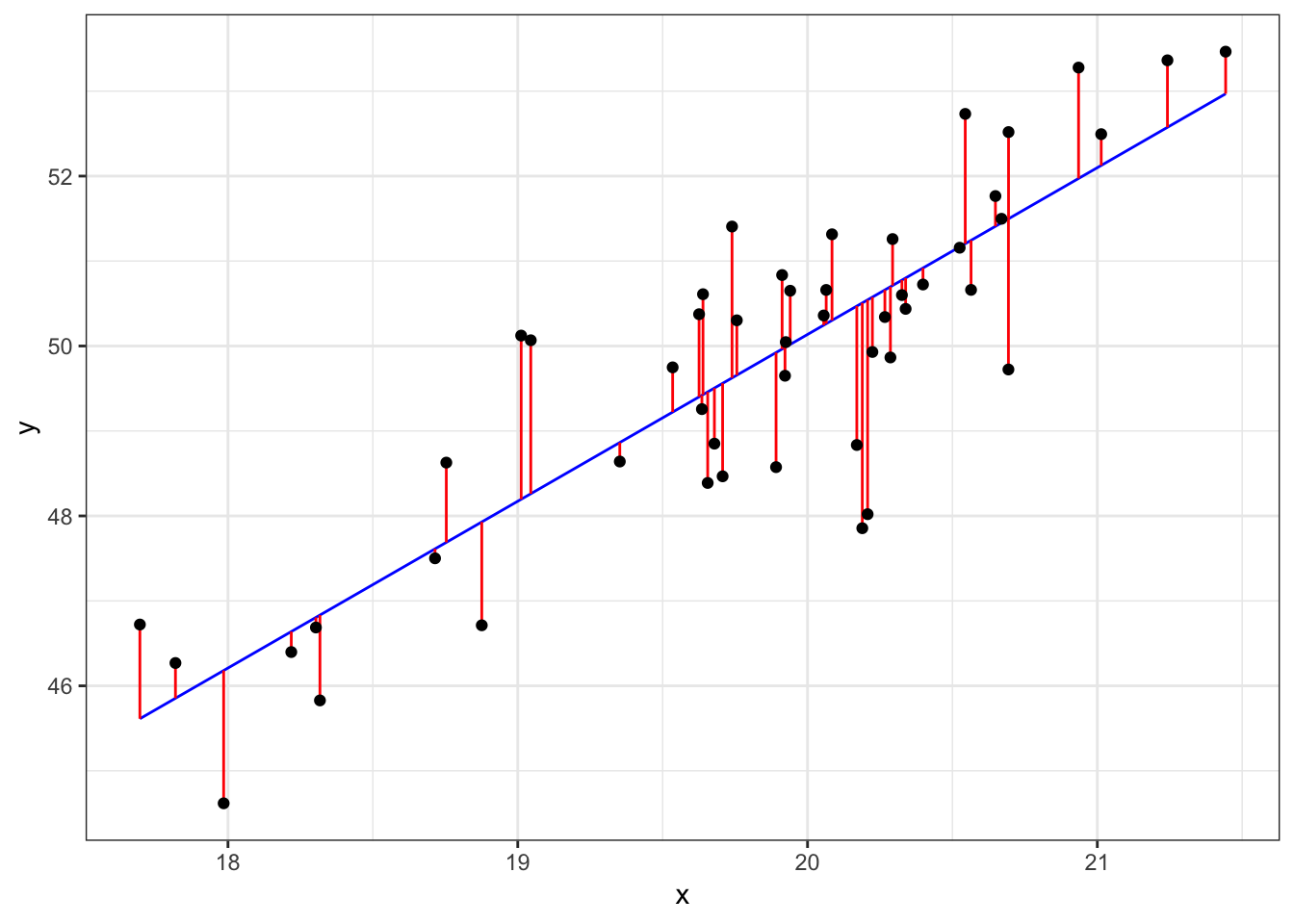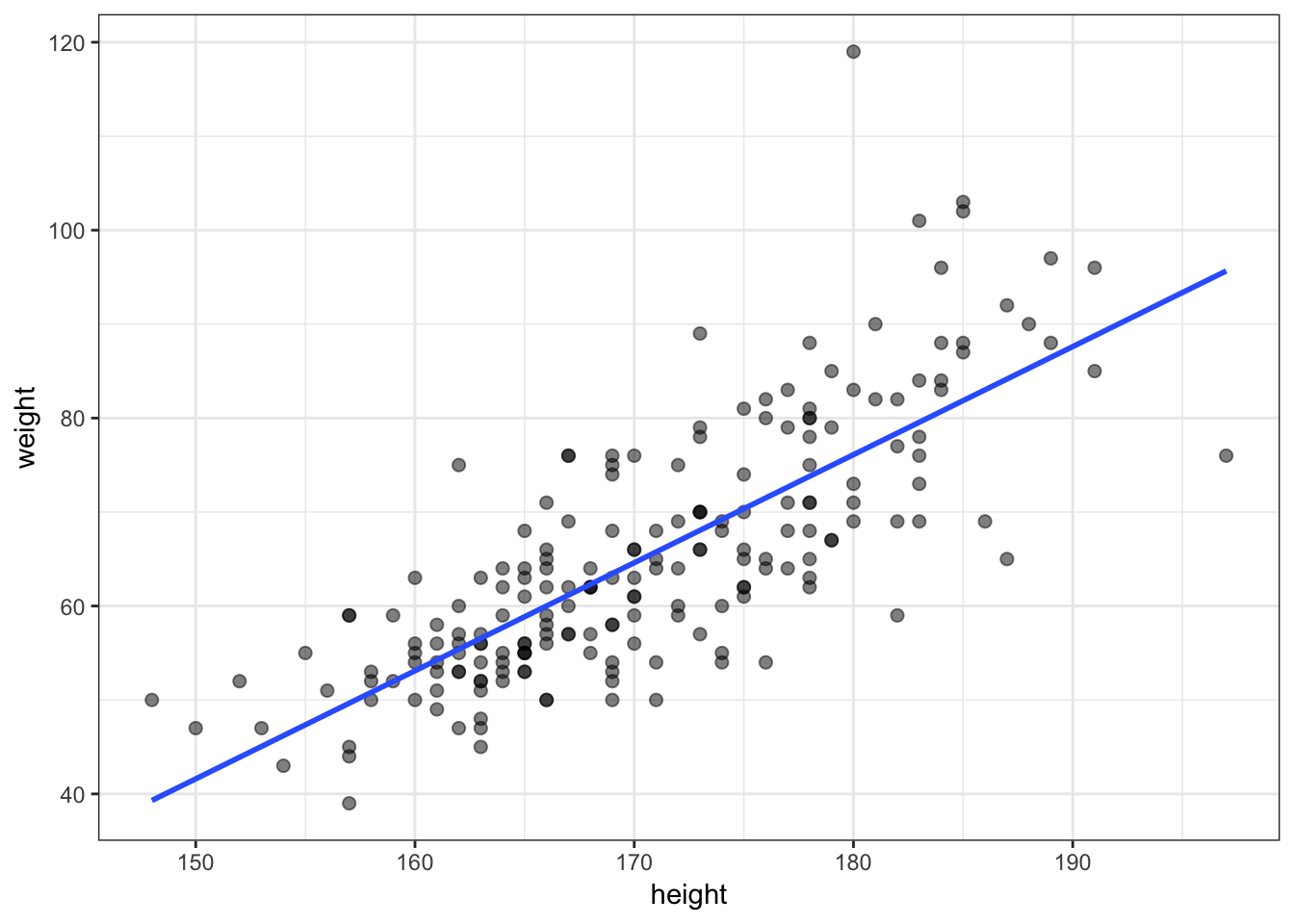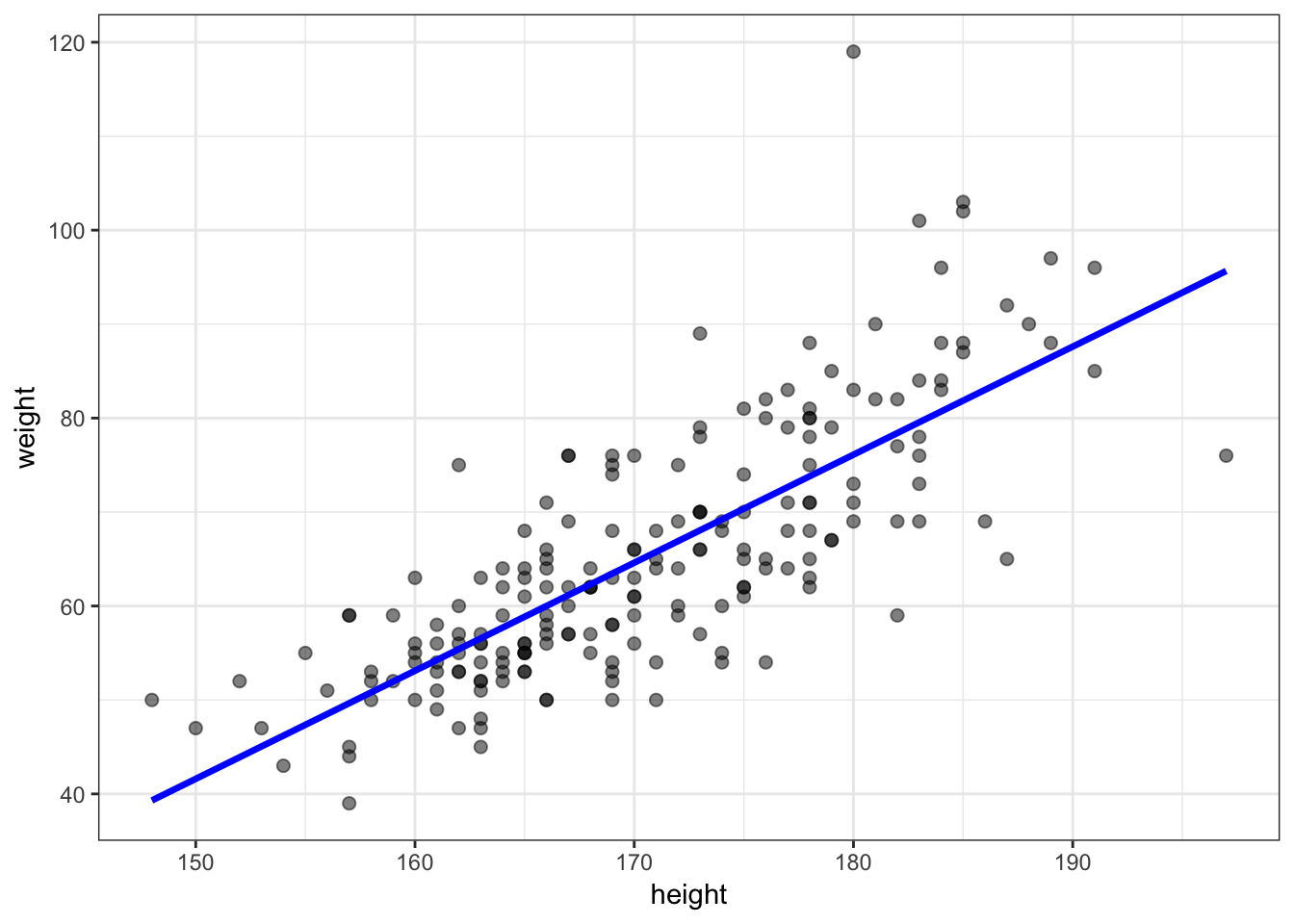56 Simple Linear Regression

56.1 Definition

For random variables $$(X_1, Y_1), (X_2, Y_2), \ldots, (X_n, Y_n)$$, simple linear regression estimates the model

$Y_i = \beta_1 + \beta_2 X_i + E_i$

where $${\operatorname{E}}[E_i] = 0$$, $${\operatorname{Var}}(E_i) = \sigma^2$$, and $${\operatorname{Cov}}(E_i, E_j) = 0$$ for all $$1 \leq i, j \leq n$$ and $$i \not= j$$.

56.2 Rationale

• Least squares linear regression is one of the simplest and most useful modeling systems for building a model that explains the variation of one variable in terms of other variables.

• It is simple to fit, it satisfies some optimality criteria, and it is straightforward to check assumptions on the data so that statistical inference can be performed.

56.3 Setup

• Suppose that we have observed $$n$$ pairs of data $$(x_1, y_1), (x_2, y_2), \ldots, (x_n, y_n)$$.

• Least squares linear regression models variation of the response variable $$y$$ in terms of the explanatory variable $$x$$ in the form of $$\beta_1 + \beta_2 x$$, where $$\beta_1$$ and $$\beta_2$$ are chosen to satisfy a least squares optimization.

56.4 Line Minimizing Squared Error

The least squares regression line is formed from the value of $$\beta_1$$ and $$\beta_2$$ that minimize:

$\sum_{i=1}^n \left( y_i - \beta_1 - \beta_2 x_i \right)^2.$

For a given set of data, there is a unique solution to this minimization as long as there are at least two unique values among $$x_1, x_2, \ldots, x_n$$.

Let $$\hat{\beta_1}$$ and $$\hat{\beta_2}$$ be the values that minimize this sum of squares.

56.5 Least Squares Solution

These values are:

$\hat{\beta}_2 = r_{xy} \frac{s_y}{s_x}$

$\hat{\beta}_1 = \overline{y} - \hat{\beta}_2 \overline{x}$

These values have a useful interpretation.

56.6 Visualizing Least Squares Line56.7 Example: Height and Weight

> ggplot(data=htwt, mapping=aes(x=height, y=weight)) +
+   geom_point(size=2, alpha=0.5) +
+   geom_smooth(method="lm", se=FALSE, formula=y~x)56.8 Calculate the Line Directly

> beta2 <- cor(htwt$height, htwt$weight) *
+                sd(htwt$weight) / sd(htwt$height)
> beta2
 1.150092
>
> beta1 <- mean(htwt$weight) - beta2 * mean(htwt$height)
> beta1
 -130.9104
>
> yhat <- beta1 + beta2 * htwt\$height

56.9 Plot the Line

> df <- data.frame(htwt, yhat=yhat)
> ggplot(data=df) + geom_point(aes(x=height, y=weight), size=2, alpha=0.5) +
+   geom_line(aes(x=height, y=yhat), color="blue", size=1.2)56.10 Observed Data, Fits, and Residuals

We observe data $$(x_1, y_1), \ldots, (x_n, y_n)$$. Note that we only observe $$X_i$$ and $$Y_i$$ from the generative model $$Y_i = \beta_1 + \beta_2 X_i + E_i$$.

We calculate fitted values and observed residuals:

$\hat{y}_i = \hat{\beta}_1 + \hat{\beta}_2 x_i$

$\hat{e}_i = y_i - \hat{y}_i$

By construction, it is the case that $$\sum_{i=1}^n \hat{e}_i = 0$$.

56.11 Proportion of Variation Explained

The proportion of variance explained by the fitted model is called $$R^2$$ or $$r^2$$. It is calculated by:

$r^2 = \frac{s^2_{\hat{y}}}{s^2_{y}}$